#has-images

### Logarithm and exponential [ edit ]

Let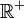be the multiplicative group of positive real numbers, and let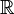be the additive group of real numbers.

The logarithm function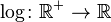satisfies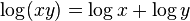for all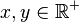, so it is a group homomorphism. The exponential function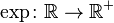satisfies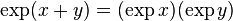for all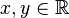, so it too is a homomorphism.

The identities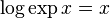and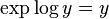show that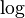and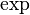are inverses of each other. Sinceis a homomorphism that has an inverse that is also a homomorphism,is an isomorphism of groups.

Becauseis an isomorphism, it translates multiplication of positive real numbers into addition of real numbers. This facility makes it possible to multiply real numbers using a ruler and a table of logarithms, or using a slide rule with a logarithmic scale.

If you want to change selection, open document below and click on "Move attachment"

Isomorphism - Wikipedia, the free encyclopedia
and exponential 1.2 Integers modulo 6 1.3 Relation-preserving isomorphism 2 Isomorphism vs. bijective morphism 3 Applications 4 Relation with equality 5 See also 6 Notes 7 References 8 Further reading 9 External links Examples <span>Logarithm and exponential Let be the multiplicative group of positive real numbers, and let be the additive group of real numbers. The logarithm function satisfies for all , so it is a group homomorphism. The exponential function satisfies for all , so it too is a homomorphism. The identities and show that and are inverses of each other. Since is a homomorphism that has an inverse that is also a homomorphism, is an isomorphism of groups. Because is an isomorphism, it translates multiplication of positive real numbers into addition of real numbers. This facility makes it possible to multiply real numbers using a ruler and a table of logarithms, or using a slide rule with a logarithmic scale. Integers modulo 6 Consider the group , the integers from 0 to 5 with addition modulo 6. Also consider the group , the ordered pairs where the x coordinates can be 0 or 1, and the